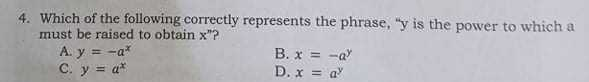### Still have math questions?

Arithmetic
Question4. Which of the following correctly represents the phrase, " $$y$$ is the power to which a must be raised to obtain $$x ^ { \prime \prime }$$ ?

A. $$y = - a ^ { x }$$ B. $$x = - a ^ { y }$$

C. $$y = a ^ { x }$$     D. $$x = a ^ { y }$$